Publication date: 05/24/2021

## Design for Fixed Blocks

Traditional screening designs require block sizes to be a power of two. However, the Custom Design platform can create designs with fixed blocks of any size.

Suppose that you want to study three factors. You can run only three trials per day and you expect substantial day-to-day variation. Consequently, you need to block your design over multiple days. Also, in this study, you are interested in estimating all two-factor interactions. In this example, you construct a design with three runs per block.

1. Select DOE > Custom Design.

2. In the Factors outline, type 3 next to Add N Factors.

3. Click Add Factor > Continuous.

4. Click Add Factor > Blocking > 3 runs per block.

The blocking factor X4 shows only one level under Values. This is because the run size is unknown at this point.

Figure 5.21 Factors Outline Showing One Block for X4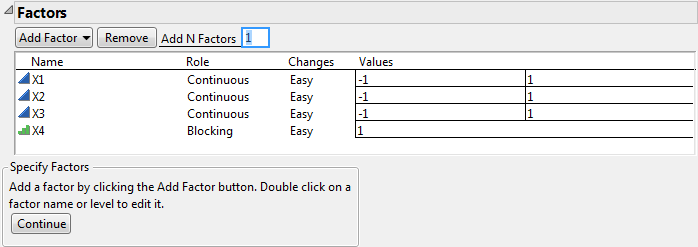5. Click Continue.

Figure 5.22 Factors Outline Showing Three Blocks for X4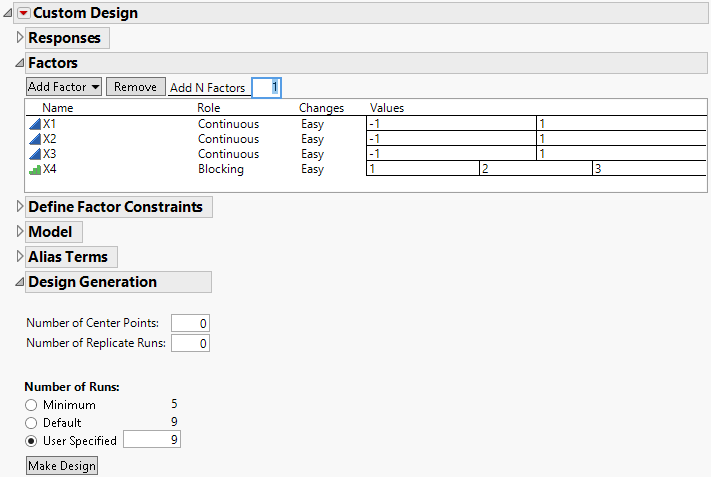The Factors outline now shows an appropriate number of blocks, calculated as the Default run size divided by the number of runs per block. For this example, the default sample size of 9 requires three blocks. The Factors outline now shows that X4 has three values, indicating the three blocks.

Note: If you specify a different number of runs, the Factors outline updates to show the appropriate number of values for the blocking factor.

6. Select the three continuous factors, X1, X2, and X3, in the Factors outline.

7. In the Model outline, click Interactions > 2nd.

Figure 5.23 Factors Outline Showing Six Blocks for X4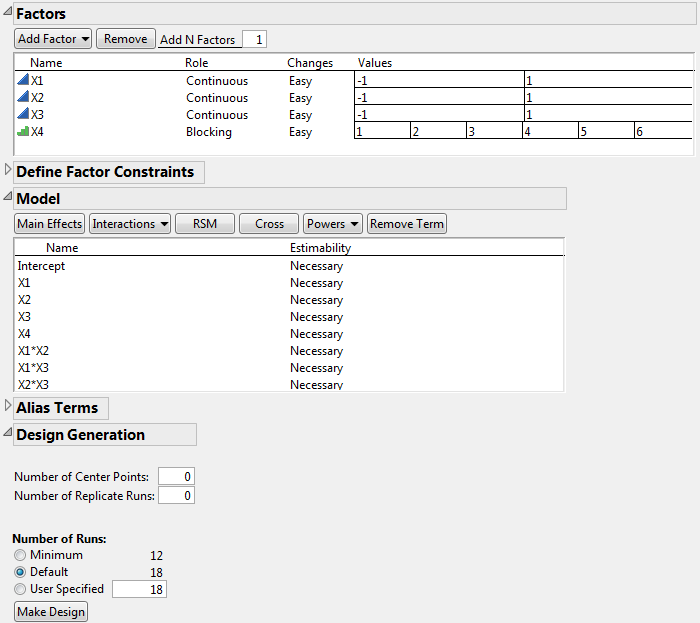The Number of Runs panel now shows that 18 is the Default run size. The Factors outline now shows six values for X4, indicating six blocks.

Note: Setting the Random Seed in step 8 and Number of Starts in step 9 reproduces the exact results shown in this example. In constructing a design on your own, these steps are not necessary.

8. (Optional) Click the Custom Design red triangle, select Set Random Seed, type 12345, and click OK.

9. (Optional) Click the Custom Design red triangle, select Number of Starts, type 5, and click OK.

10. Click Make Design.

Figure 5.24 Fixed Block Design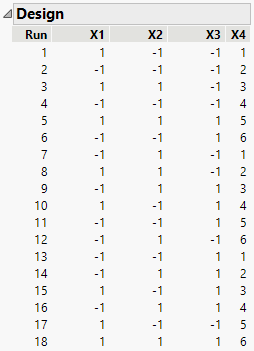In the design, look at the blocking factor, X4. The six blocks are represented. When you conduct your experiment, each day you will run three trials, where X4 = 1 on the first day, X4 = 2 on the second day, and so on. So you want the design table to randomize the trials within blocks. In the Output Options panel, the Randomize within Blocks option is already selected for Run Order.

11. Click Make Table.

Figure 5.25 Design Table for Fixed Block Design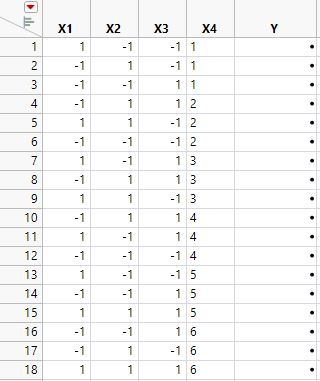The rows in the design table are grouped by each day’s runs. This design enables you to estimate the block effect, all main effects, and two-factor interactions.